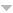# Publications

Found 172 results
[ Author] Title Type Year
Filters: First Letter Of Last Name is R  [Clear All Filters]
R
A surface with canonical map of degree 24. International Journal of Mathematics. 2017;28(6).Edit
Some bidouble planes with $p_g=q=0$ and $4\leq K^2\leq 7$. International Journal of Mathematics. 2015;26(5).
Cuspidal quintics and surfaces with $p_g=0,$ $K^2=3$ and 5-torsion. LMS Journal of Computation and Mathematics. 2016;19(1):42-53.
New surfaces with $K^2=7$ and $p_g=q\leq 2$. The Asian Journal of Mathematics. In Press.Edit
On the construction of complex algebraic surfaces. Vol 38, 39 2017.Edit
Hyperelliptic surfaces with $K^2 < 4\chi - 6$. Osaka Journal of Mathematics. 2015;52(4):929-947.Edit
Explicit Schoen surfaces. Algebraic Geometry. In Press.Edit
A surface with $q=2$ and canonical map of degree $16$. Michigan Mathematical Journal. 2017;66(1):99-105.
New canonical triple covers of surfaces. Proceedings of the American Mathematical Society. 2015;143(11):4647-4653.
Pneumococcal pneumonia-are the new severity scores more accurate in predictingadverse outcomes? [Pneumonia pneumocócica-serão os novos scoresmais precisos a prever eventos desfavoráveis?]. Revista Portuguesa de Pneumologia. 2013;19:252-259.Edit
The semaphore codes attached to a Turing machine via resets and their various limits. Internat. J. Algebra Comput.. 2016;26(4):675-704.Edit
Boolean Representations of Simplicial Complexes and Matroids. Vol Springer Monographs in Mathematics Springer 2015.Edit
A new notion of vertex independence and rank for finite graphs. Internat. J. Algebra Comput.. 2015;25(1-2):123-167.Edit
Random walks on semaphore codes and delay de Bruijn semigroups. Internat. J. Algebra Comput.. 2016;26(4):635-673.Edit
Turing machines and bimachines. Theoret. Comput. Sci.. 2008;400:182-224.Edit